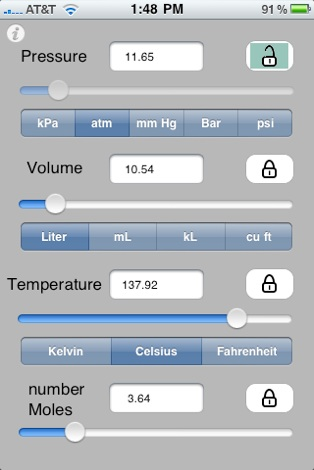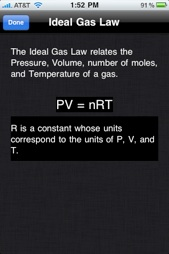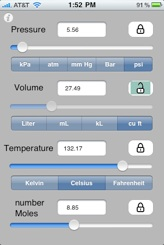Ideal Gas LawThis awesome calculator lets you compute and modify the ideal gas equation PV=nRT using any of the 4 parameters as the free parameter and with different units for P, V, and T.

The convenient sliders let you see the effect of changing one of the independent parameters on the dependent one giving you a very natural understanding of how the relationship works.

Students, educators, researchers, and those interested in computational chemistry will all appreciate the convenience of seeing the computation recomputed for different units with a simple touch of the selector bar.

Using the Ideal Gas Law Calculatorsupport and info: a2s2@mac.com